# Diabetic Retinopathy Detection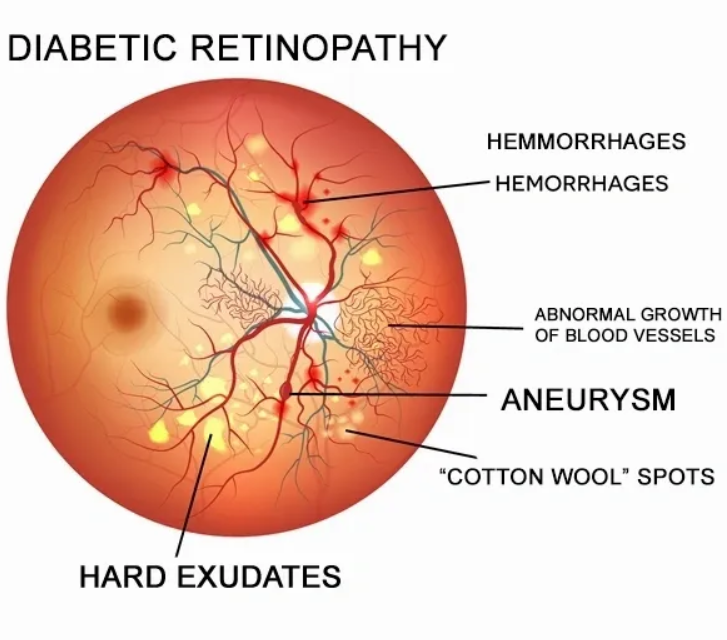In this article, I worked on Diabetic Retinopathy Detection that was a competition on Kaggle.

This competition contains 4 different labels, these are

0 - No DR

1 - Mild

2 - Moderate

3 - Severe

4 - Proliferative DR

When we check the images, we can realize that we should apply some operations before training. These operations will be used for destroying the black areas, making clear blood vessels.

In order to do these steps, first I will upload images and check them.

Above, I read the first 20 images, because today there are 3662 in the training dataset, I just want to take a look at the first step.

As is seen, the second image has a huge black area, we don't want it, cause it avoids learning, and also it makes images smaller. Therefore I will crop these black areas.

BUT for now, I will go on just the first image, after complete these steps in the first image, I will define a function that makes apply these steps to all datasets.

To crop the image, first I need to convert it to GRAYSCALE because I will apply THRESHOLD and in order to apply that, the image should be GRAY.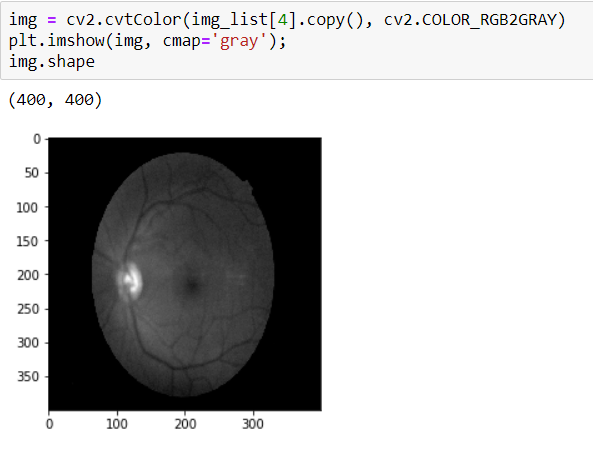Before THRESHOLD, I need to apply Median Blurring because my aim is destroying the black area and applying the Blurring operation, it makes the contour of image clarity.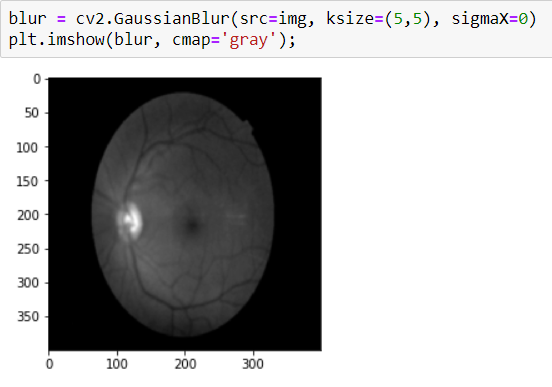Well, now I am ready to apply THRESHOLD, it is so simple: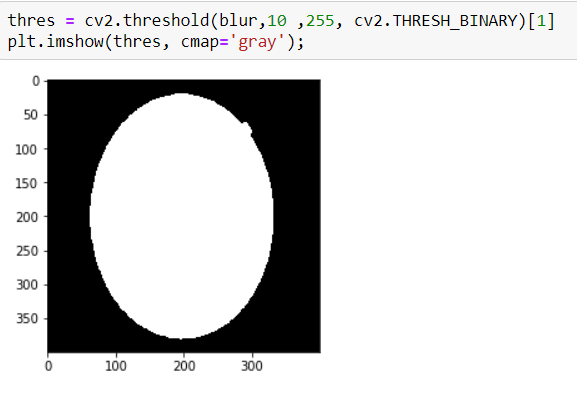Now I have an image that has a clear contour.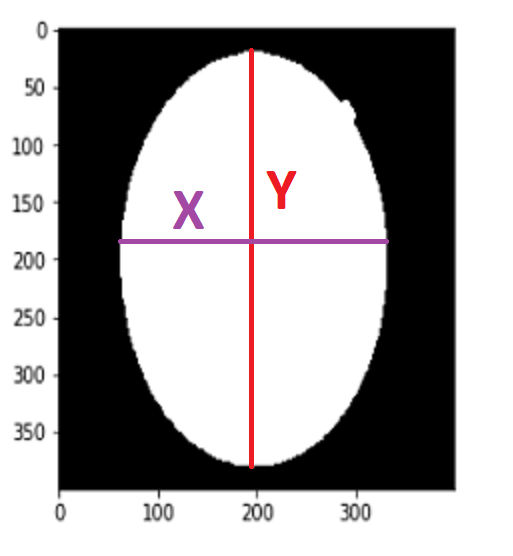To find Contour I will use a method call cv2.findContours() When we use this method, it returns an array

After getting the list of coordinates, I will find max and min values for X and Y axes.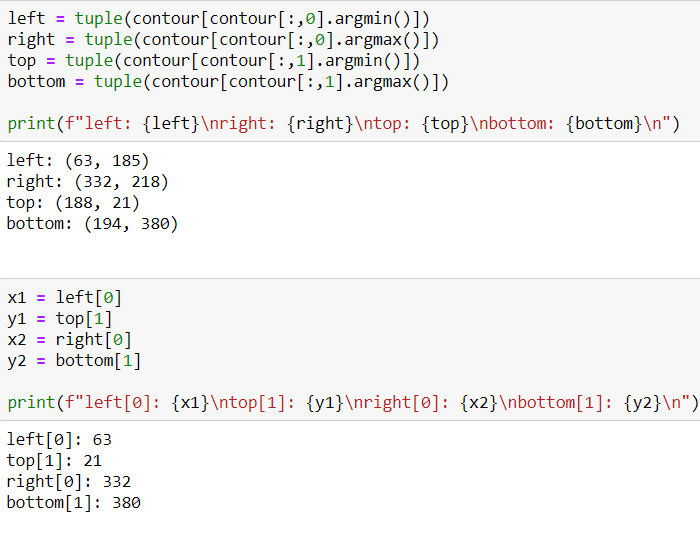Now I have coordinates for cropping operations. So I can crop it. To crop an image there is no method actually, I just use slicing.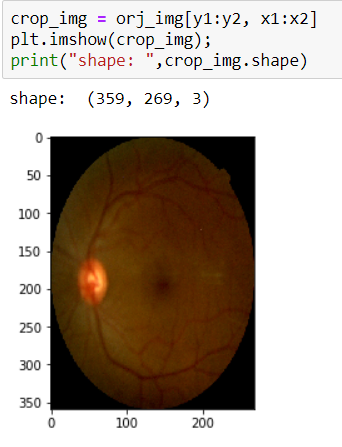The image has been cropped! BUT as you can see, its sizes are changed due to crop. So now, I need to resize it again.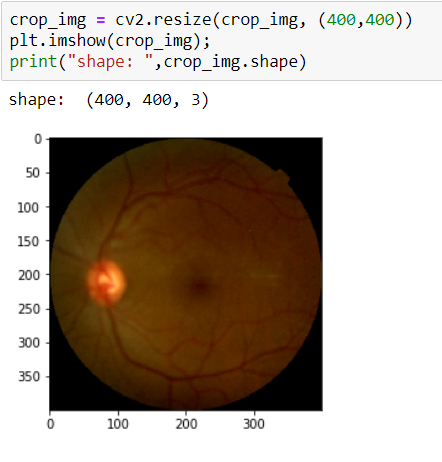That's all.

Now We are in the important part called CLAHE

Contrast-Limited Adaptive Histogram Equalization (CLAHE) is a variant of adaptive histogram equalization in which the contrast amplification is limited.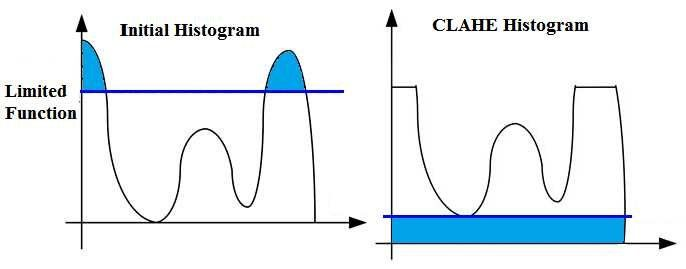In order to apply, CLAHE image should be LAB format, for that, I will convert the image according to this method cv2.COLOR_RGB2LAB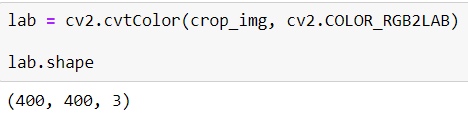I applied this method easily but as you can see it has 3 channel, they are:

• L => Lightning
• A => Green - Red
• B => Blue - Yellow

In these channels, I need to reach L layers, for that, I will use this method cv2.split() and as a result of this method, it returns 3 parameters (L, A, B)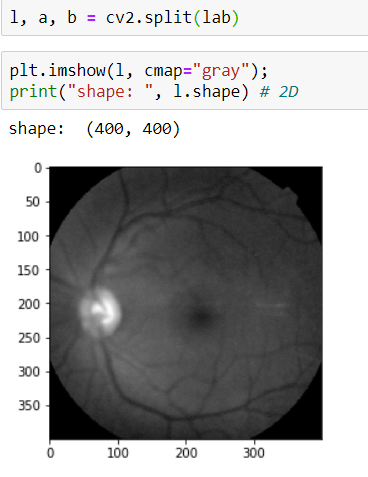Above the image, it has 2 sizes now.

Now I can apply CLAHE. But before that, first I want to show their Histograms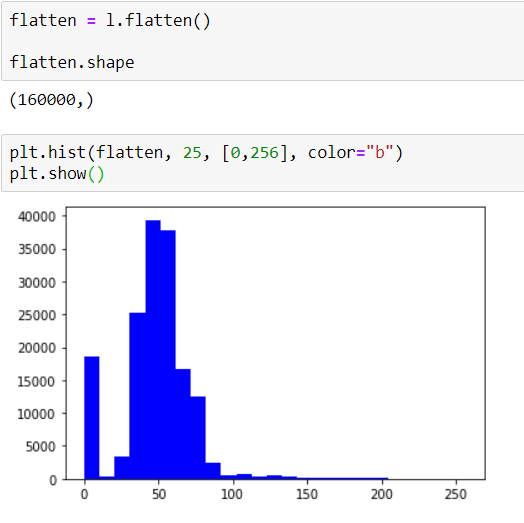as you can see, it doesn't have a Symmetrical Distribution.

BUT now I will apply CLAHE and then you can check the new Histogram.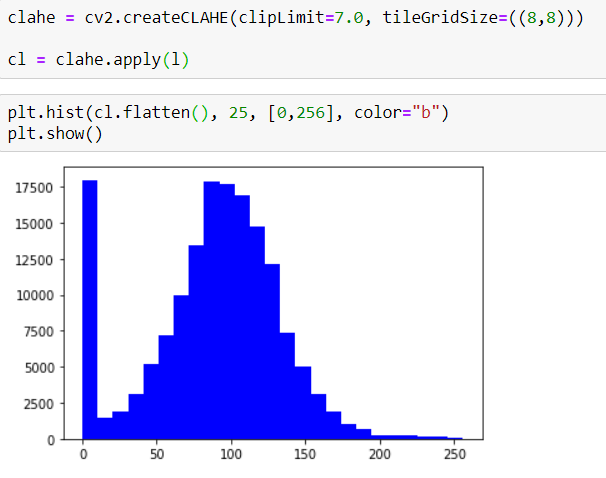now the image has a more Symmetrical Distribution.

If the Histogram Graphs doesn't have a meaning, you can check the before/after images: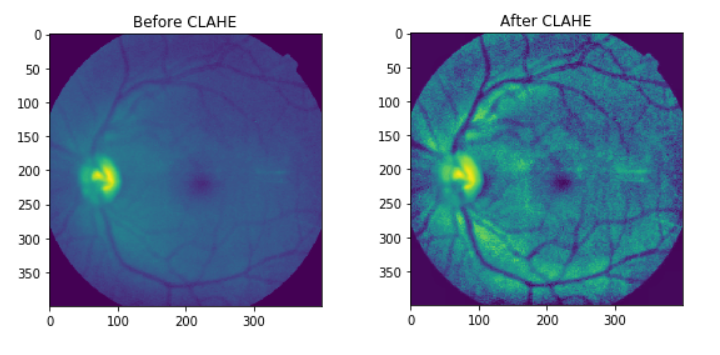Now that I have applied CLAHE I can move on to other steps,

The other step is MERGING, before I split L, A, B channels, now it's time to MERGE them. In order to merge them, I will use cv2.merge()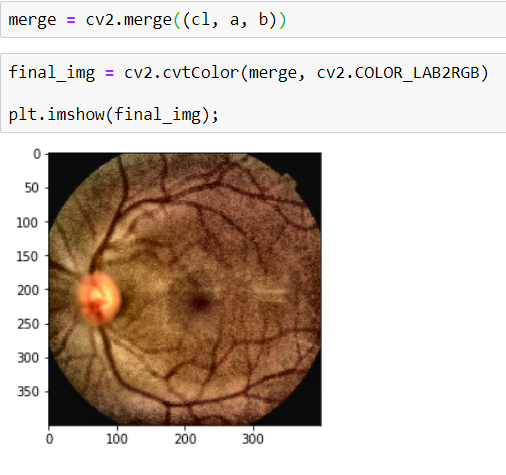Now I have a really good looking image but actually, I shouldn't say good looking because it has a noise (Salt-Peper). The best way to destroy them is by using BLURRING OPERATION*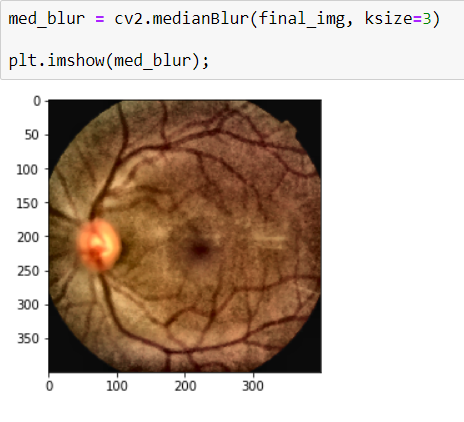It is a little better now.

The other important, even the most important step is making blood vessels more clearly.

To do that, First I will apply BLURRING with a huge kernel size because it makes the image more blur, just the blood vessels will appear, after that, I will have a background that has appeared blood vessels.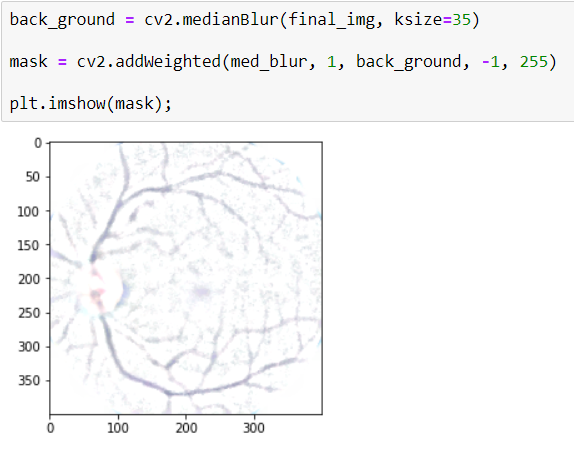Here, I want to explain some parameters.

``````cv2.addWeighted(src1=med_blur, alpha=1, src2=back_ground, beta=-1, gamma=255)

``````

I want to use BLUR IMAGE 100%, for that aplha=1
also, beta=-1 means, reverse all values of pixels. Gamma=255 is for reverse image color. as you can see it turned white. And thus, only blood vessels appear.

Now I will apply to mask to the image. For that, I will use cv2.bitwise_and() We can say that it merges two images.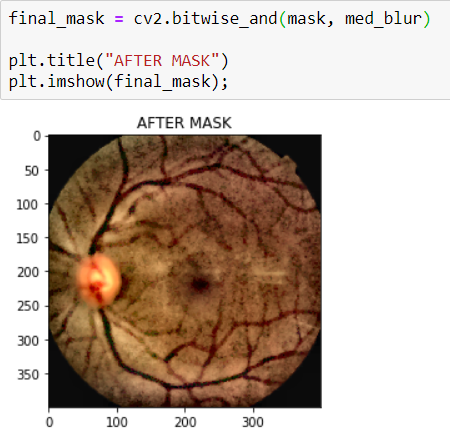If we compare before/after versions: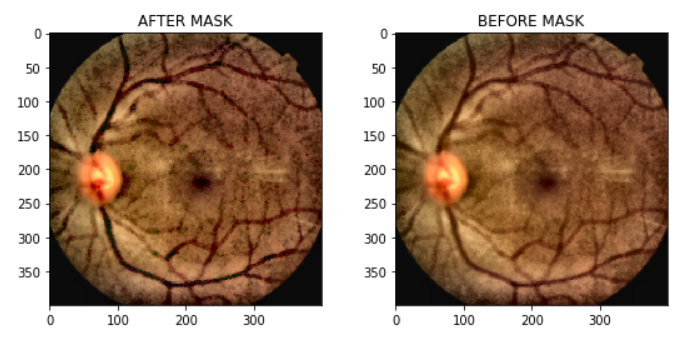After these steps, I can see that works! now I will define a function in a python file because I used jupyter notebook for that I can see the result immediately. Also in next, I will implement Transfer Learning Models. For that Pycharm is my favorite IDE.

After applied function:

``````from tqdm import tqdm

path = "data\\datasets\\"
files = os.listdir(path + "train_images")

def preprocessing(files):
"""
This function returns images prepared for training
"""
img_list = []
for i in tqdm(files):
image = cv2.imread(path + 'train_images\\' + i)
image = cv2.resize(image, (400, 400))
image = cv2.cvtColor(image, cv2.COLOR_BGR2RGB)

copy = image.copy()
copy = cv2.cvtColor(copy, cv2.COLOR_RGB2GRAY)
blur = cv2.GaussianBlur(copy, (5, 5), 0)

thresh = cv2.threshold(blur, 10, 255, cv2.THRESH_BINARY)

# CONTOUR DETECTION
contour = cv2.findContours(thresh.copy(), cv2.RETR_EXTERNAL, cv2.CHAIN_APPROX_SIMPLE)
contour = contour
contour = contour[:, 0, :]

# GET COORDINATES
x1 = tuple(contour[contour[:, 0].argmin()])
y1 = tuple(contour[contour[:, 1].argmin()])
x2 = tuple(contour[contour[:, 0].argmax()])
y2 = tuple(contour[contour[:, 1].argmax()])

#Crop Images Again to Destroy Black Area
x = int(x2 - x1) * 4 // 50
y = int(y2 - y1) * 5 // 50

# THRES FOR CROPPED IMAGES
copy2 = image.copy()
if x2 - x1 > 100 and y2 - y1 > 100:
copy2 = copy2[y1 + y: y2 - y, x1 + x: x2 - x]
copy2 = cv2.resize(copy2, (400, 400))

# LAB
lab = cv2.cvtColor(copy2, cv2.COLOR_RGB2LAB)
l, a, b = cv2.split(lab)

# CLAHE - Contrast-Limited Adaptive Histogram Equalization
clahe = cv2.createCLAHE(clipLimit=5.0, tileGridSize=((8, 8)))
cl = clahe.apply(l)

# MERGING LAB
merge = cv2.merge((cl, a, b))
final_img = cv2.cvtColor(merge, cv2.COLOR_LAB2RGB)
med_blur = cv2.medianBlur(final_img, 3)
back_gorund = cv2.medianBlur(final_img, 37)

img_list.append(final)

return img_list

img_list = preprocessing(files=files)

``````

Now, I can transform my labels to One Hote Encoding, For that: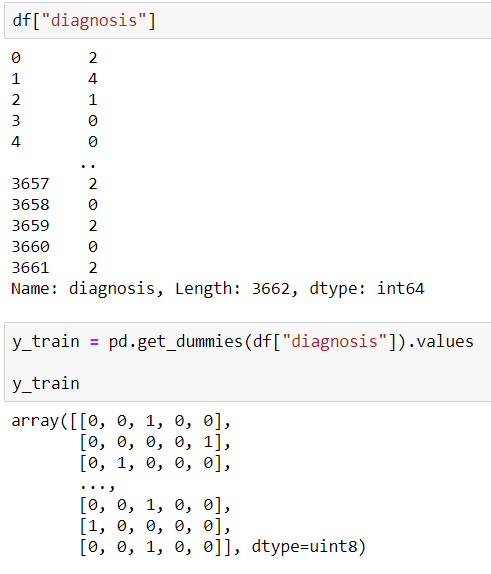But here there is a really important situation. Images are not Multi-Class they are Multi-Label In the same image, there are more probabilities. I mean if the patient's scala is 3 (it means "Severe") also the patient has 0,1 and 2 scalas. Because these symptoms correlated with each self.
*Summary If the patient has a scala 2 problem, we can see scala 0, scala 1, and scala 2, as told before they are correlated with each self.
In this situation labels shoul be like that:

• If scala 4 => [1, 1, 1, 1, 1]
• If scala 3 => [1, 1, 1, 1, 0]
• If scala 2 => [1, 1, 1, 0, 0]
• If scala 1 => [1, 1, 0, 0, 0]

In order to convert that I will use a simple for loop and I will use np.logical_or() method. This method computes the truth value of x1 OR x2 element-wise.

``````np.logical_or(1,1)
>> True

np.logical_or(0,1)
>> True

np.logical_or(0,0)
>> False
``````

To convert to Multi-Label: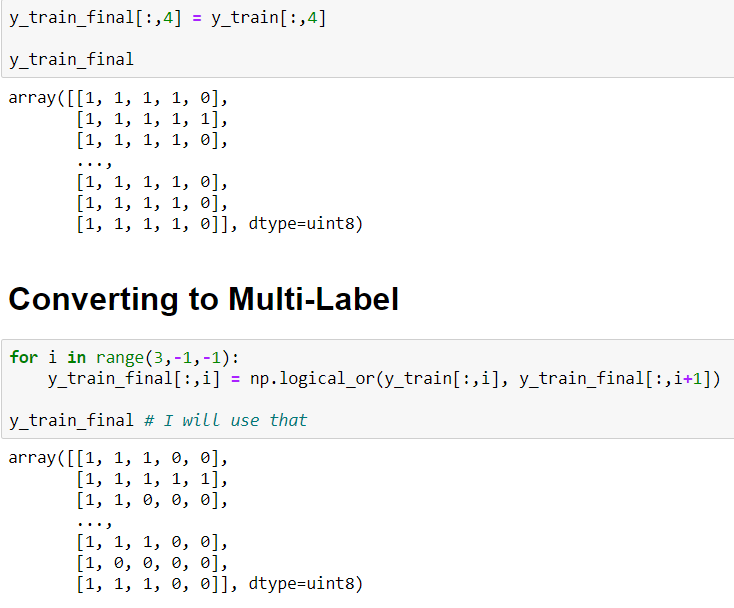After this steps: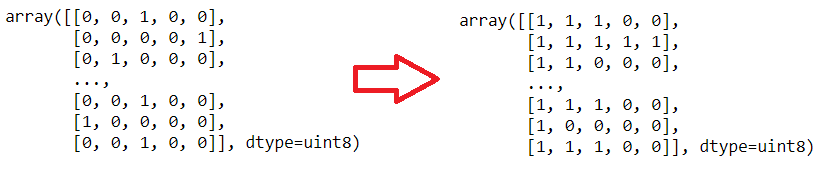And now I am ready to define the transfer learning model but first I need to transform my images into arrays. It is so simple with this method np.array()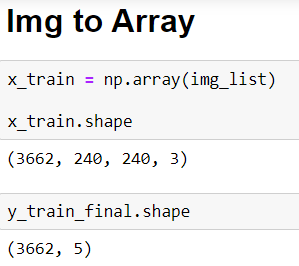So, one more step to define the model, it's time to split data: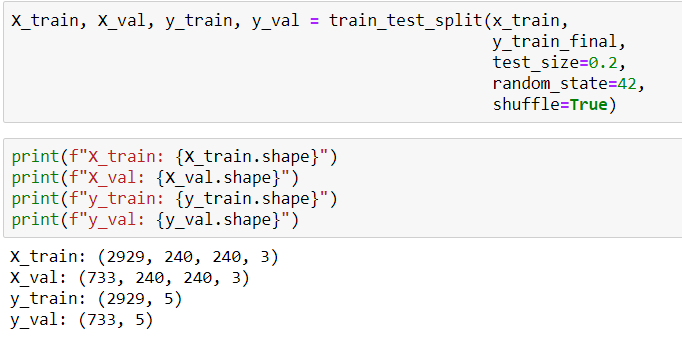Now I will define a Generator to generate more images for training. Because the model has to face images in different ways. It makes the model better!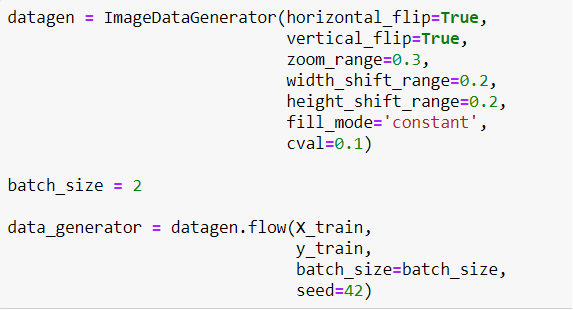Maybe you realize that I didn't use rescale. Because the transfer learning model called EfficientNetB5 I will use, makes images rescale itself. So I don't need to do that.

Now it's time to define the transfer learning model. But first, the model must be downloaded:

``````pip install git+https://github.com/qubvel/segmentation_models
``````

After installation I will create Neural Network: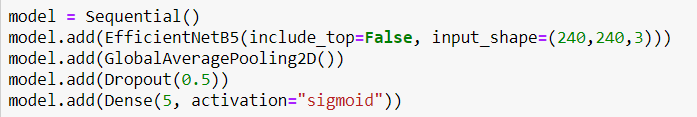In the transfer learning model called EfficientNetB5 I chose include_top=False because I don't want to use the last part of layers, also I've changed the input size as (240,240,3) first it was (400,400,3) but on my pc, it doesn't work because of the RAM :)

Then I compile my model like that and also I define a callback for Learning Rate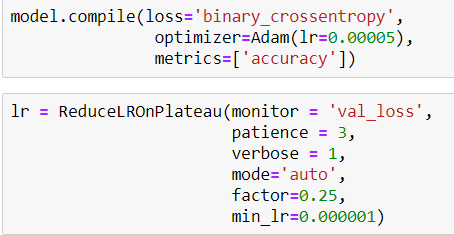Finally I am in the training step: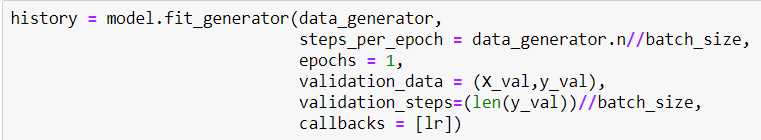Unfortunately my pc doesn't have enough RAM so because of that I can not train my model :( but I will figure out that as soon as possible.